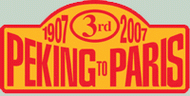Фото

/ start from Tyumen to Yekaterinburg /

close

1 . Tyumen.2 . Tyumen.3 . Tyumen.4 . Tyumen.5 . Tyumen.6 . Tyumen.7 . Tyumen.8 . Tyumen.9 . Tyumen.10 . Tyumen.11 . Tyumen.12 . Tyumen.13 . Tyumen.14 . Tyumen.15 . Tyumen.16 . Tyumen.17 . Tyumen.18 . Tyumen.19 . Tyumen.20 . Tyumen.21 . Tyumen.22 . Tyumen.23 . Tyumen.24 . Tyumen.25 . Tyumen.26 . Tyumen.27 . Tyumen.28 . Tyumen.29 . Tyumen.30 . Tyumen.31 . Tyumen.32 . Tyumen.33 . Tyumen.34 . Tyumen.35 . Tyumen.36 . Tyumen.37 . Tyumen.38 . Tyumen.39 . Tyumen.40 . Tyumen.41 . Tyumen.42 . Tyumen.43 . Tyumen.44 . Tyumen.45 . Tyumen.46 . Tyumen.47 . Tyumen.48 . Tyumen.49 . Tyumen.50 . Tyumen.51 . Tyumen.52 . Tyumen.53 . Tyumen.top   close